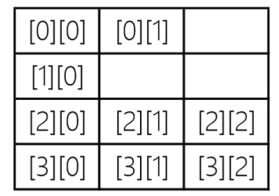# Java不规则数组详解

TK 2020-09-18 11:22:35 java常见问答 8802

`int intArray[][] = {{1,2}, {11}, {21,22,23}, {31,32,33}};````int intArray[][] = new int[]; //先初始化高维数组为4
// 逐一初始化低维数组
intArray = new int;
intArray = new int;
intArray = new int;
intArray = new int;``````import java.util.Arrays;
public class HelloWorld
{
public static void main(String[] args)
{
int intArray[][] = new int[]; // 先初始化高维数组为4
// 逐一初始化低维数组
intArray = new int;
intArray = new int;
intArray = new int;
intArray = new int;
// for循环遍历
for (int i = 0; i < intArray.length; i++)
{
for (int j = 0; j < intArray[i].length; j++)
{
intArray[i][j] = i + j;
}
}
// for-each循环遍历
for (int[] row: intArray)
{
for (int column: row)
{
System.out.print(column);
// 在元素之间添加制表符，
System.out.print('\t');
}
// 一行元素打印完成后换行
System.out.println();
}
System.out.println(intArray); // 发生运行期错误
}
}```

Java不规则数组就给你简单的介绍到这里了，更多java基础知识，可以继续来奇Q工具网进行了解哦。

java二维数组，获取整列元素详解

Java一维数组，获取单个元素实例分享

java数组，数组是什么?特点有哪些?